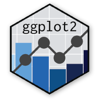Show Sidebar Hide Sidebar# geom_point in ggplot2

How to make a scatter chart in ggplot2. Examples of scatter charts and line charts with fits and regressions.

### New to Plotly?

Plotly's R library is free and open source!
You can set up Plotly to work in online or offline mode.
We also have a quick-reference cheatsheet (new!) to help you get started!

### Version Check

Version 4 of Plotly's R package is now available!
Check out this post for more information on breaking changes and new features available in this version.

library(plotly)
packageVersion('plotly')

##  '4.9.1'


### Scatter Chart

library(plotly)

set.seed(955)
# Make some noisily increasing data
dat <- data.frame(cond = rep(c("A", "B"), each=10),
xvar = 1:20 + rnorm(20,sd=3),
yvar = 1:20 + rnorm(20,sd=3))

p <- ggplot(dat, aes(x=xvar, y=yvar)) +
geom_point(shape=1)      # Use hollow circles

p <- ggplotly(p)

p


### Liner Regression w/ smooth

library(plotly)

set.seed(955)
# Make some noisily increasing data
dat <- data.frame(cond = rep(c("A", "B"), each=10),
xvar = 1:20 + rnorm(20,sd=3),
yvar = 1:20 + rnorm(20,sd=3))

p <- ggplot(dat, aes(x=xvar, y=yvar)) +
geom_point(shape=1) +    # Use hollow circles
geom_smooth(method=lm)   # Add linear regression line

p <- ggplotly(p)

p


library(plotly)

set.seed(955)
# Make some noisily increasing data
dat <- data.frame(cond = rep(c("A", "B"), each=10),
xvar = 1:20 + rnorm(20,sd=3),
yvar = 1:20 + rnorm(20,sd=3))

p <- ggplot(dat, aes(x=xvar, y=yvar)) +
geom_point(shape=1) +    # Use hollow circles
geom_smooth(method=lm,   # Add linear regression line

p <- ggplotly(p)

p


### Loess Smoothed Fit

library(plotly)

set.seed(955)
# Make some noisily increasing data
dat <- data.frame(cond = rep(c("A", "B"), each=10),
xvar = 1:20 + rnorm(20,sd=3),
yvar = 1:20 + rnorm(20,sd=3))

p <- ggplot(dat, aes(x=xvar, y=yvar)) +
geom_point(shape=1) +    # Use hollow circles
geom_smooth()            # Add a loess smoothed fit curve with confidence region
# > geom_smooth: method="auto" and size of largest group is less than 1000, so using loess.
# Use 'method = x' to change the smoothing method.

p <- ggplotly(p)

p


### Constrained Slope

library(plotly)

set.seed(1234)

n <- 20

x1 <- rnorm(n); x2 <- rnorm(n)
y1 <- 2 * x1 + rnorm(n)
y2 <- 3 * x2 + (2 + rnorm(n))
A <- as.factor(rep(c(1, 2), each = n))
df <- data.frame(x = c(x1, x2), y = c(y1, y2), A = A)
fm <- lm(y ~ x + A, data = df)

p <- ggplot(data = cbind(df, pred = predict(fm)), aes(x = x, y = y, color = A))
p <- p + geom_point() + geom_line(aes(y = pred))

p <- ggplotly(p)

p


Inspire by Stack Overflow

### Stat Summary

library(plotly)

hist <- data.frame(date=Sys.Date() + 0:13, counts=1:14)
hist <- transform(hist, weekday=factor(weekdays(date), levels=c('Monday', 'Tuesday', 'Wednesday', 'Thursday', 'Friday', 'Saturday', 'Sunday')))

p <- ggplot(hist, aes(x=weekday, y=counts, group=1)) +
geom_point(stat='summary', fun.y=sum) +
stat_summary(fun.y=sum, geom="line")

p <- ggplotly(p)

p


Inspire by Stack Overflow

### Control Line Order

library(plotly)

dat <- data.frame(x = sample(1:10), y = sample(1:10), order = sample(1:10))
p <- ggplot(dat[order(dat$order),], aes(x, y)) + geom_point() + geom_text(aes(y = y + 0.25,label = order)) + geom_path() p <- ggplotly(p) p  ### Horizontal Line w/ Segment library(plotly) p <- ggplot(mtcars,aes(mpg,qsec))+geom_point() + geom_segment(aes(x=15,xend=20,y=18,yend=18)) p <- ggplotly(p) p  Inspired by Stack Overflow ### Add Points library(plotly) df <- data.frame(time=as.factor(c(1,1,2,2,3,3,4,4,5,5)), value=as.numeric(c(7, 8, 9, 10, 10, 11, 10.5, 11.4, 10.9, 11.6)), side=as.factor(c("E","F","E","F","E","F","E","F","E","F"))) p <- ggplot(df, aes(time, value, group=side, colour=side)) + geom_line(size=1) p <- p + geom_point() p <- ggplotly(p) p  ### Add Regression w/ Abline library(plotly) set.seed(1) x <- 1:10 dd <- rbind(data.frame(x=x,fac="a", y=x+rnorm(10)), data.frame(x=2*x,fac="b", y=x+rnorm(10))) coef <- lm(y~x:fac, data=dd)$coefficients
p <- qplot(data=dd, x=x, y=y, color=fac)+
geom_abline(slope=coef["x:faca"], intercept=coef["(Intercept)"])+
geom_abline(slope=coef["x:facb"], intercept=coef["(Intercept)"])

p <- ggplotly(p)

p


Inspired by Stats Exchange